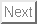Up: J.E. Sonier's M.Sc. Thesis Previous: Bibliography

# Some Remarks About The Fitting Program

The computer time allocated to calculating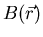of Eq. (3.9), is significant enough that it is practical to avoid the large sum over reciprocal lattice vectors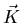for each iteration in the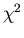-minimization procedure. Consequently, a Taylor series expansion around an initial value of the magnetic penetration depth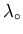was employed in the actual fitting program: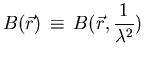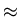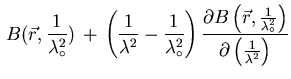+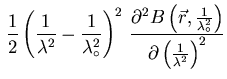(1)

so that,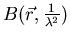is determined by expanding about the initial point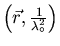. In this way,is calculated by summing over the reciprocal lattice vectors[in Eq. (3.9)], only once for an initial set of parameters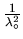and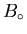, whereis the initial value of the average field. It is not necessary to expand about. This is because changes in the average field merely shift the field distribution along the field (or frequency) axis. In the fitting process, field shifts in excess of a conservative value of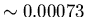T were not permitted before the program was stopped, the initial parameters changed, and the data refitted.

Table A.1: Accuracy of the Taylor series expansion used in the fitting program.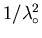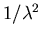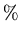Error inError inat theat the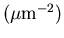vortex core. saddle point. 50 50 0.00 0.00 50 49 0.16 0.14 50 48 0.31 0.27 50 47 0.47 0.40 50 46 0.62 0.53 50 45 0.78 0.67 50 44 0.93 0.80 50 43 1.08 0.93 50 30 3.01 2.65 50 20 4.43 3.96 50 10 5.78 5.25 50 0 7.07 6.54

Table A.1 shows the accuracy of the Taylor series assuming an average magnetic field 0.5T and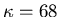. The error in using Eq. (A.1), expressed as a percentage of the exact calculation ofusing Eq. (3.9), is shown forcalculated at the vortex core and at a saddle point. The results show that even large changes in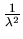give a good approximation for. In a typical fit,is calculated in excess of 150,000times, so that using a Taylor series greatly diminishes the time required to fit.Up: J.E. Sonier's M.Sc. Thesis Previous: Bibliography
Jess H. Brewer
2001-09-28